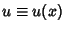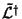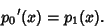Given a differential equation(1)

whereand, the Adjoint Operatoris defined by(2)

In order for the operator to be self-adjoint, i.e.,(3)

the second terms in (1) and (2) must be equal, so(4)

This also guarantees that the third terms are equal, since(5)

so (2) becomes(6)(7)

The Legendre Differential Equation and the equation of Simple Harmonic Motion are self-adjoint, but the Laguerre Differential Equation and Hermite Differential Equation are not.

A nonself-adjoint second-order linear differential operator can always be transformed into a self-adjoint one using Sturm-Liouville Theory. In the special case, (7) gives(8)(9)(10)(11)

whereis a constant of integration.

A self-adjoint operator which satisfies the Boundary Conditions(12)

is automatically a Hermitian Operator.

Arfken, G. Self-Adjoint Differential Equations.'' §9.1 in Mathematical Methods for Physicists, 3rd ed. Orlando, FL: Academic Press, pp. 497-509, 1985.